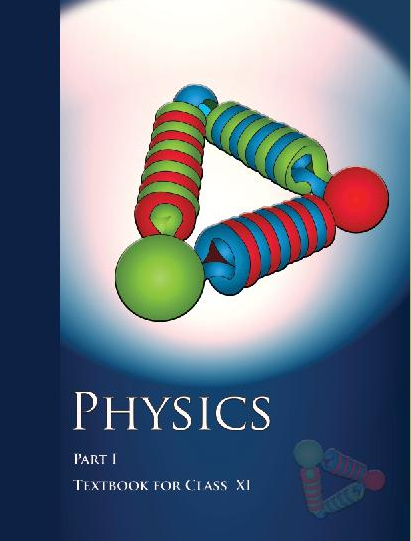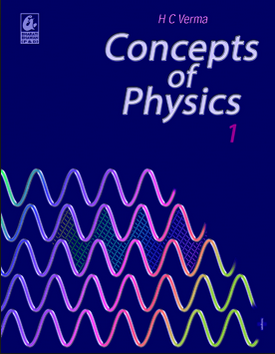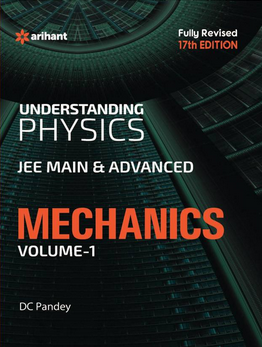# Work Energy and Power (Weightage 3%)   Share

## How to prepare Work Energy and Power for JEE Main 2020 2020

Overview of the chapter

To study this chapter efficiently first you have to understand all the definitions and terminologies, given the chapters and try to interpret them in a very logical way. You must be good at basic laws like the law of energy conservation and theorems like a work-energy theorem Because you might get conceptual questions based on these laws And theorems. You will get various real-life application based problems on the concept like a work-energy theorem, law of energy conservation, Collision, Power.
For example, let's have a look question which is based on real-life activities and was asked in jee main 2016 from this chapter.

Q-  A person who is trying to lose weight by burning his fats lifts a mass of 10 kg 1000 times up to a height of 1 m.  And also assume that the potential energy lost each time he lowers the mass is dissipated.  By considering the work done only when the weight is lifted up then tell How much fat will he use up? ( If Fat supplies 3.8× J of energy on burning 1 kg fat which is converted to mechanical energy with a 20% efficiency rate. )

To solve these questions you first find work done by the person in lifting the weight
Which is equal to W=mgh
Which is equal to  E

Then find Total fat burn by dividing W by E
Which is equal to

## How to Prepare

First, you should have good command over the concepts of Work Power and Energy and you should also know how to apply them well at the time of the entrance exam or while solving questions. Entrance 360 will help you to understand each concept from this chapter since it provides you theory, questions with solutions and video lectures on each important concepts. For each concept practice enough problems so that you are through with the concept. Take mock test and practice previous year questions. Through practice, you will come to know how to approach a question. There are direct formulas that can be applied to certain questions.

To Solve Work Power Energy Problems

• .Master the concept of Kinematics, Laws of motion, Centre Of Mass, Impulse, and Momentum. This will help you to understand applying force in the problems.
• After reading problems you must define a system and then identify all the forces in the system and energy going in and out.
• You should try to balance the kinetic energy, potential energy and the work done for the given problem. Pay special attention to questions on springs and try similar questions from HCV and  Entrance360 site.
• You must know all the formulas from this chapter, and use these formulas to find unknown variables.

## Tips

• Make a plan to prepare for the chapter and Stick to a Timetable, Entrance 360 knockout series will help you make customized time table according to available time for preparation.
• Go through all NCERT basics, the NCERT textbooks are very important and most advisable for understanding theory and it mostly covers the full syllabus.
• Study the concept first and then start solving the question. Don't go through question directly without knowing a concept.
• Solve previous year question paper.
• Take a practice test.
• Use smart methods to solve questions.

Books

For Work Power and Energy chapter concepts in NCERT is enough and practice questions which are asked in previous questions and you can follow other standard books available for JEE preparation like Concepts of Physics (H. C. Verma) and Understanding Physics by D. C. Pandey (Arihant Publications). You don't have to study the whole book to understand the concept from this chapter because Entrance 360 will provide you exact page number and line number of these books where you will get these concepts to read.

### Topics from Work Energy and Power

• Work done by force (15 concepts)
• Kinetic and Potential Energy (6 concepts)
• Potential energy of a spring (1 concepts)
• Work Energy Theorem (2 concepts)
• Conservation of energy (3 concepts)
• Power (4 concepts)
• Elastic and Inelastic Collisions (11 concepts)
• Work (5 concepts)
• Energy and Power (8 concepts)
• Collision (7 concepts)

### Important Books for Work Energy and Power

•••Exams
Articles
Questions